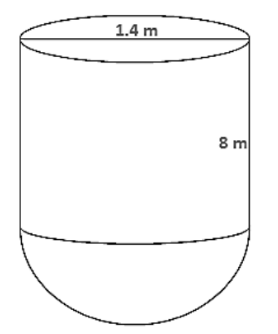# A storage tank consists of a circular cylinder with a hemisphere adjoined on either end.

Question:

A storage tank consists of a circular cylinder with a hemisphere adjoined on either end. If the external diameter of the cylinder be 1.4 m and its length is 8 m, find the cost of painting it on the outside at the rate of Rs.10 per m2

Solution:

Diameter of a cylinder = 1.4 m

Therefore radius of cylinder = 1.42 = 0.7 m

Height of cylinder = 8 m

Therefore surface area of tank $=2 \pi r h+2 \pi r^{2}$

$=2 \times 22 / 7 \times 0.7 \times 8+2 \times 22 / 7 \times(0.7)^{2}$

$=176 / 5+77 / 25=38.28 \mathrm{~m}^{2}$

Now cost of $1 \mathrm{~m}^{2}=\operatorname{Rs} .10$

Therefore cost of $38.28 \mathrm{~m}^{2}=$ Rs. $382.80$